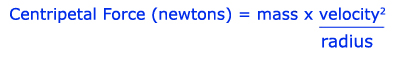## Circular Motion & Centripetal Force

From Newton’s first law of motion it is known that an object will remain stationary, or keep moving at constant velocity in a straight line unless acted upon by an unbalanced force. When an object moves in a circular path its direction is changing all the time therefore according to Newton’s first law there must be an unbalanced force acting upon it all the time.

When an object moves in a circle although its speed is constant the direction is continuously changing. Therefore its velocity is continuously changing as velocity is speed in a particular direction. The changing velocity in time means the object is accelerating all the time.

The resultant force which causes this acceleration is the centripetal force.

The centripetal force always acts toward the centre of the circle.

The centripetal force is determined from the following equation:If a ball is tied to the end of a strong string and swung in a circle, the ball accelerates towards the centre of the circle. The centripetal force which causes the inwards acceleration is from the tension in the string caused by the person’s hand pulling the string. If the string breaks there is no longer a resultant force acting on the ball, so it will continue its motion in a straight line at constant speed.The centripetal force required to make an object perform circular motion increases in the following cases:

• If the mass of the object increases.
• If the velocity of the object increases.
• If the radius of the circle decreases.

The above points are evident when considering the equation for centripetal force.